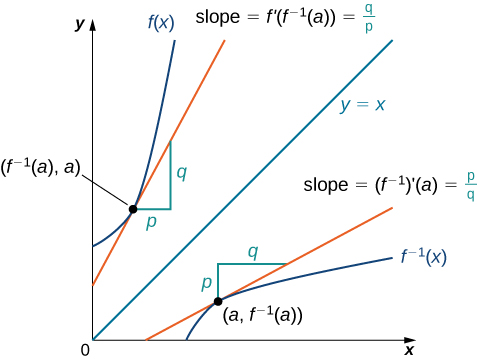# 3.7 Derivatives of inverse functions

 Page 1 / 3
• Calculate the derivative of an inverse function.
• Recognize the derivatives of the standard inverse trigonometric functions.

In this section we explore the relationship between the derivative of a function and the derivative of its inverse. For functions whose derivatives we already know, we can use this relationship to find derivatives of inverses without having to use the limit definition of the derivative. In particular, we will apply the formula for derivatives of inverse functions to trigonometric functions. This formula may also be used to extend the power rule to rational exponents.

## The derivative of an inverse function

We begin by considering a function and its inverse. If $f\left(x\right)$ is both invertible and differentiable, it seems reasonable that the inverse of $f\left(x\right)$ is also differentiable. [link] shows the relationship between a function $f\left(x\right)$ and its inverse ${f}^{-1}\left(x\right).$ Look at the point $\left(a,{f}^{-1}\left(a\right)\right)$ on the graph of ${f}^{-1}\left(x\right)$ having a tangent line with a slope of ${\left({f}^{-1}\right)}^{\prime }\left(a\right)=\frac{p}{q}.$ This point corresponds to a point $\left({f}^{-1}\left(a\right),a\right)$ on the graph of $f\left(x\right)$ having a tangent line with a slope of ${f}^{\prime }\left({f}^{-1}\left(a\right)\right)=\frac{q}{p}.$ Thus, if ${f}^{-1}\left(x\right)$ is differentiable at $a,$ then it must be the case that

${\left({f}^{-1}\right)}^{\prime }\left(a\right)=\frac{1}{{f}^{\prime }\left({f}^{-1}\left(a\right)\right)}.$The tangent lines of a function and its inverse are related; so, too, are the derivatives of these functions.

We may also derive the formula for the derivative of the inverse by first recalling that $x=f\left({f}^{-1}\left(x\right)\right).$ Then by differentiating both sides of this equation (using the chain rule on the right), we obtain

$1={f}^{\prime }\left({f}^{-1}\left(x\right)\right)\left({f}^{-1}{\right)}^{\prime }\left(x\right)\right).$

Solving for $\left({f}^{-1}{\right)}^{\prime }\left(x\right),$ we obtain

${\left({f}^{-1}\right)}^{\prime }\left(x\right)=\frac{1}{{f}^{\prime }\left({f}^{-1}\left(x\right)\right)}.$

We summarize this result in the following theorem.

## Inverse function theorem

Let $f\left(x\right)$ be a function that is both invertible and differentiable. Let $y={f}^{-1}\left(x\right)$ be the inverse of $f\left(x\right).$ For all $x$ satisfying ${f}^{\prime }\left({f}^{-1}\left(x\right)\right)\ne 0,$

$\frac{dy}{dx}=\frac{d}{dx}\left({f}^{-1}\left(x\right)\right)={\left({f}^{-1}\right)}^{\prime }\left(x\right)=\frac{1}{{f}^{\prime }\left({f}^{-1}\left(x\right)\right)}.$

Alternatively, if $y=g\left(x\right)$ is the inverse of $f\left(x\right),$ then

$g\left(x\right)=\frac{1}{{f}^{\prime }\left(g\left(x\right)\right)}.$

## Applying the inverse function theorem

Use the inverse function theorem to find the derivative of $g\left(x\right)=\frac{x+2}{x}.$ Compare the resulting derivative to that obtained by differentiating the function directly.

The inverse of $g\left(x\right)=\frac{x+2}{x}$ is $f\left(x\right)=\frac{2}{x-1}.$ Since ${g}^{\prime }\left(x\right)=\frac{1}{{f}^{\prime }\left(g\left(x\right)\right)},$ begin by finding ${f}^{\prime }\left(x\right).$ Thus,

${f}^{\prime }\left(x\right)=\frac{-2}{{\left(x-1\right)}^{2}}\phantom{\rule{0.2em}{0ex}}\text{and}\phantom{\rule{0.2em}{0ex}}{f}^{\prime }\left(g\left(x\right)\right)=\frac{-2}{{\left(g\left(x\right)-1\right)}^{2}}=\frac{-2}{{\left(\frac{x+2}{x}-1\right)}^{2}}=-\frac{{x}^{2}}{2}.$

Finally,

${g}^{\prime }\left(x\right)=\frac{1}{{f}^{\prime }\left(g\left(x\right)\right)}=-\frac{2}{{x}^{2}}.$

We can verify that this is the correct derivative by applying the quotient rule to $g\left(x\right)$ to obtain

${g}^{\prime }\left(x\right)=-\frac{2}{{x}^{2}}.$

Use the inverse function theorem to find the derivative of $g\left(x\right)=\frac{1}{x+2}.$ Compare the result obtained by differentiating $g\left(x\right)$ directly.

${g}^{\prime }\left(x\right)=-\frac{1}{{\left(x+2\right)}^{2}}$

## Applying the inverse function theorem

Use the inverse function theorem to find the derivative of $g\left(x\right)=\sqrt{x}.$

The function $g\left(x\right)=\sqrt{x}$ is the inverse of the function $f\left(x\right)={x}^{3}.$ Since ${g}^{\prime }\left(x\right)=\frac{1}{{f}^{\prime }\left(g\left(x\right)\right)},$ begin by finding ${f}^{\prime }\left(x\right).$ Thus,

${f}^{\prime }\left(x\right)=3{x}^{3}\phantom{\rule{0.2em}{0ex}}\text{and}\phantom{\rule{0.2em}{0ex}}{f}^{\prime }\left(g\left(x\right)\right)=3{\left(\sqrt{x}\right)}^{2}=3{x}^{2\text{/}3}.$

Finally,

${g}^{\prime }\left(x\right)=\frac{1}{3{x}^{2\text{/}3}}=\frac{1}{3}{x}^{-2\text{/}3}.$

Find the derivative of $g\left(x\right)=\sqrt{x}$ by applying the inverse function theorem.

$g\left(x\right)=\frac{1}{5}{x}^{\text{−}4\text{/}5}$

From the previous example, we see that we can use the inverse function theorem to extend the power rule to exponents of the form $\frac{1}{n},$ where $n$ is a positive integer. This extension will ultimately allow us to differentiate ${x}^{q},$ where $q$ is any rational number.

A Function F(X)=Sinx+cosx is odd or even?
neither
David
Neither
Lovuyiso
f(x)=1/1+x^2 |=[-3,1]
apa itu?
fauzi
determine the area of the region enclosed by x²+y=1,2x-y+4=0
Hi
MP
Hi too
Vic
hello please anyone with calculus PDF should share
Which kind of pdf do you want bro?
Aftab
hi
Abdul
can I get calculus in pdf
Abdul
How to use it to slove fraction
Hello please can someone tell me the meaning of this group all about, yes I know is calculus group but yet nothing is showing up
Shodipo
You have downloaded the aplication Calculus Volume 1, tackling about lessons for (mostly) college freshmen, Calculus 1: Differential, and this group I think aims to let concerns and questions from students who want to clarify something about the subject. Well, this is what I guess so.
Jean
Im not in college but this will still help
nothing
how can we scatch a parabola graph
Ok
Endalkachew
how can I solve differentiation?
with the help of different formulas and Rules. we use formulas according to given condition or according to questions
CALCULUS
For example any questions...
CALCULUS
v=(x,y) وu=(x,y ) ∂u/∂x* ∂x/∂u +∂v/∂x*∂x/∂v=1
what is the procedures in solving number 1?
review of funtion role?
for the function f(x)={x^2-7x+104 x<=7 7x+55 x>7' does limx7 f(x) exist?
find dy÷dx (y^2+2 sec)^2=4(x+1)^2
Integral of e^x/(1+e^2x)tan^-1 (e^x)
why might we use the shell method instead of slicing
fg[[(45)]]²+45⅓x²=100
find the values of c such that the graph of f(x)=x^4+2x^3+cx^2+2x+2
anyone to explain some basic in calculus
I can
DebdootBy Dionne MahaffeyBy Sandy YamaneBy Janet ForresterBy JavaChamp TeamBy OpenStaxBy OpenStaxBy Cath YuBy OpenStaxBy OpenStaxBy John Gabrieli